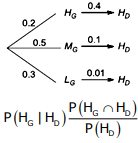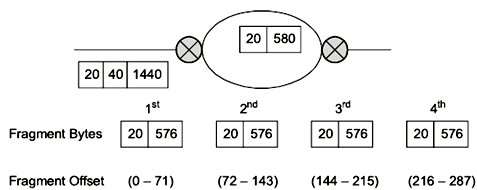# GATE Solved Paper 2017-19 - GATE 2018

• A

50• B

150• C

20• D

60• Option : A
• Explanation :
Node that receives the packet to transmit will carrier-sense the medium for 5 units. Any packet which arrives within 5 unit will be sensed and keep the channel busy. Given that Signal speed is 10 meter/time.
Which means in 5 unit of time packet can travel 50 meters in max, that allow Q to successfully avoid the collision.
So, option (A) is correct.

 Item Number Weight in (kgs) Value in Rupees) 1 10 60 2 7 28 3 4 20 4 2 24
The task is to pick a subset of these items such that their total weight is not more than 11 kgs and their total value is maximized. Moreover, no item may be split. The total value of items picked by an optimal algorithm is denoted by A greedy algorithm sorts the items by their value-to-weight ratios in descending order and packs them greedily, starting from the first item in the ordered list. The total value of items picked by the greedy algorithm is denoted by Vgreedy.
The value of Vopt − Vgreedy is ______ .

Note – Numerical Type question

• A

8• B

60• C

16• D

44• Option : C
• Explanation :  Item No. Weight Value Value/Weight 1 10 60 6 2 7 28 4 3 4 20 5 4 2 24 12
After Sorting  Item No. Weight Value Value/Weight 1 2 24 12 2 10 60 6 3 4 20 5 4 7 28 4
First, we have to Pick item_1 (Value weight ratio is highest).
After that Second highest is item_1. but cannot be picked because of its weight.
Now item_3 shall be picked. item_2 cannot be included because of its weight.
Therefore, overall profit by Vgreedy = 20+24 = 44
Hence, Vopt – Vgreedy = 60-44 = 16
So, answer is 16.

Note – Numerical Type question

• A

10230• B

10240• C

1023• D

1024• Option : A
• Explanation :
Count(1024, 1024) will be called and value of x will be deducted by x/2. ‘x’ will be printed 10 times. On count=10, value of x will become 1.
For every recursively for every y=1023 and count() is called 10times for each y. So, 1023 x 10 = 10230
Answer is 10230.

The following table gives the conditional probabilities for Delhi’s temperature given Guwahati’s temperature.

 HD MD LD HG 0.40 0.48 0.12 MG 0.10 0.65 0.25 LG 0.01 0.50 0.49
Consider the first row in the table above. The first entry denotes that if Guwahati has high temperature (HG) then the probability of Delhi also having a high temperature (HD) is 0.40; i.e., P(HD ∣ HG) = 0.40. Similarly, the next two entries are P(MD ∣ HG) = 0.48 and P(LD ∣ HG) = 0.12. Similarly for the other rows.
If it is known that P(HG) = 0.2, P(MG) = 0.5, and P(LG) = 0.3, then the probability (correct to two decimal places) that Guwahati has high temperature given that Delhi has high temperature is _______ .

Note – Numerical Type question.

• A

0.6015• B

0.6150• C

0.5016• D

0.6510• Option : A
• Explanation : The condition probability table given is  HD MD LD HG 0.40 0.48 0.12 MG 0.10 0.65 0.25 LG 0.01 0.50 0.49

P(HG) = 0.2
P(MG) = 0.5
P(LG) = 0.3
Drawing the tree diagram for HD we get,From diagram, P(HG ∩ HD) = 0.2x0.4
P(HD) = 0.2x0.4+0.5x0.1+0.3x0.01 = 0.133
Required probability,
P(HG | HD) = (0.2x0.4)/0.133 = 0.60

• A

0• B

72• C

144• D

216• Option : C
• Explanation :Related Quiz.
GATE 2018